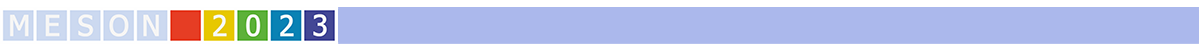#MESON2023

Jun 22 – 27, 2023
Auditorium Maximum
Europe/Warsaw timezone

## Dynamical generation of axial-vector mesons

Jun 22, 2023, 5:50 PM
20m
Medium lecture hall (B) (Auditorium Maximum)

Parallel

### Speaker

Hyun-Chul Kim (Inha University)

### Description

We present recent results on the dynamical generation of the $a_1$, $h_1$, and $b_1$ axial-vector mesons. We demonstrate the emergence of the $a_1$ and $h_1$ mesons from the $\pi\rho$ scattering process, based on the coupled-channel formalism with the $\pi\rho$ and $K\bar{K}^*$ ($\bar{K}K^*$) channels. This is achieved by constructing kernel amplitudes using the effective Lagrangian and computing the coupled integral equation for $\pi\rho$ scattering. By performing the partial-wave expansion, we explicitly show that the $a_1(1260)$ meson arises as a $K\bar{K}^*$ molecular state, generated only by including the $K\bar{K}^*$ ($\bar{K}K^*$) channel. The pole position of the $a_1$ meson is determined to be at $\sqrt{s_R}=(1170.7-i173.0)$ MeV. We also investigate four different $h_1$ mesons by coupling additional channels such as $\eta\omega$ and $\eta\phi$, and find that $h_1(1415)$ is strongly coupled to the $K\bar{K}^*$ and $\eta\phi$ channels, indicating its significant strange quark content. Additionally, we report a novel observation that the $b_1$ meson has a two-pole structure that arises from $\pi\omega$ scattering, with the interference of these two-pole resonances resulting in the appearance of $b_1(1235)$.

### Primary author

Hyun-Chul Kim (Inha University)

### Co-author

Samson Clymton (Inha University)